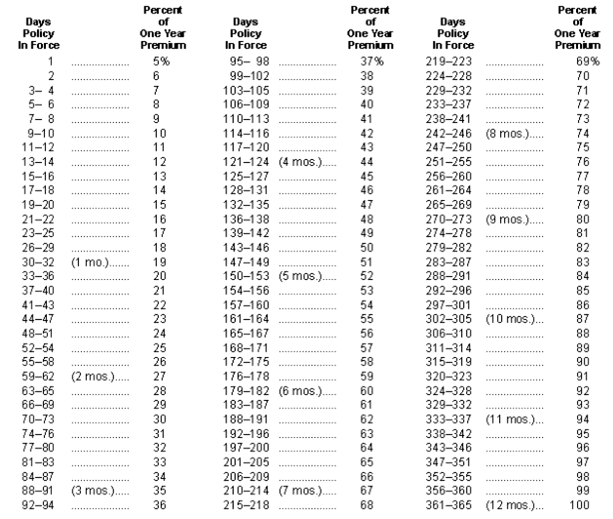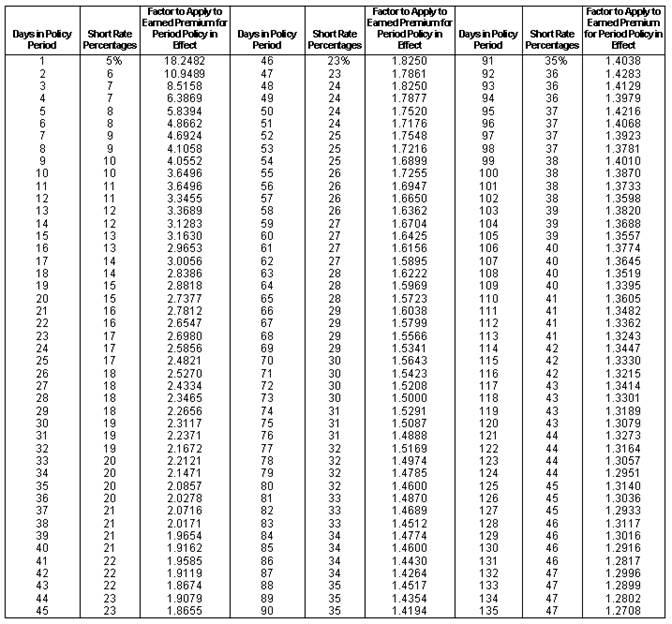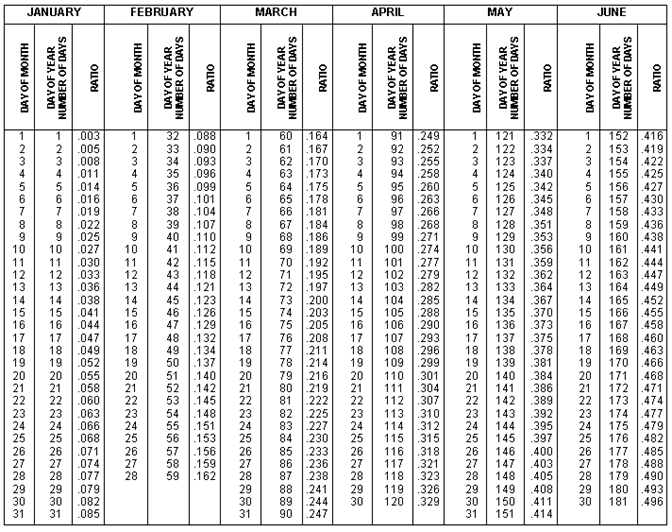### Appendix B - Cancellation Tables and Examples

 Revision History Type Effective Date Circular Notes Original 04/01/2016 C-16-6 North Carolina Basic Manual for Workers Compensation and Employer Liability - Digital Edition

Refer to Rule 3-A-3

SHORT RATE CANCELLATION TABLE FOR A TERM OF ONE YEARSHORT RATE CANCELLATION TABLESHORT RATE CANCELLATION TABLE (CONT’D)SHORT RATE CANCELLATION TABLE (CONT’D)PRO RATA CANCELLATION TABLEPRO RATA CANCELLATION TABLE (CONT’D)CANCELLATION EXAMPLES

The following examples have been created and apply to the Cancellation tables outlined in Rule 3-A-3.

Sample Policy Information

- Policy Effective Date:  1/1/X4

- Policy Expiration Date: 1/1/X5 (365 day term)

- Policy Cancellation Date:  7/5/X4 (in effect 185 days)

- Actual Payroll:  \$300,000 (reflecting 1/1/X4 to 7/5/X4)

- Manual Rate:  \$2

- Experience Modification:  0.95

- Expense Constant:  \$250

- Terrorism Charge of .01

- Catastrophe (other than Certified Acts of terrorism) charge of .01

Pro Rata Cancellation Example

- Pro Rata number of days ratio = 0.507

1. Payroll extended for full policy term = \$55,500*365/185 = \$109,500

2. Full policy term premium before experience rating = \$109,500 / 100 x \$2 = \$2,190

3. Full policy term Modified Premium = \$2,190*.95 = \$2,081

4. Pro-Rata modified premium = \$2,081 * 0.507 = \$1,055

5. Pro-Rata portion of expense constant = 0.507 * \$250 = \$127

6. Terrorism = (\$55,500/100 * .01) = \$6

7. Catastrophe= (\$55,500/100 * .01) = \$6

8. Total Pro Rata Premium for cancelled policy = (\$1,055 + \$127 + \$6 + \$6) = \$1,194

Short Rate percentage cancellation example

- Short Rate percentage for 185 days = 61%

1. Payroll extended for full policy term = \$55,500*365/185 = \$109,500

2. Full policy term premium before experience rating = \$109,500 / 100 x \$2 = \$2,190

3. For a 365 day policy, extended # of days = # of days policy in effect = 185

4. Short rate premium = \$2,190 * .61 = \$1,336

5. Short rate modified premium = \$1,336 * 0.95 = \$1,269

6. Less premium discount (first \$5,000 @ 0%) = \$0

7. Short rate portion of expense constant = \$250 * .61 = \$153

8. Terrorism = (\$55,500/100 * .01) = \$6

9. Catastrophe= (\$55,500/100 * .01) = \$6

10. Total premium for cancelled policy = \$1,269 + \$153 + \$6 + \$6 = \$1,434

Short Rate factor cancellation example

- Short Rate Factor for 185 days = 1.2035

1. Actual premium = \$55,500/100 * \$2 = \$1,110

2. Short rate charge = \$1,110 * .2035 = 226

3. Short rate manual premium = \$1,110 + \$226 = \$1,336

4. Short rate modified premium = \$1,336 * .95 = \$1,269

5. Less premium discount (first \$5,000 @ 0%) = \$0

6. Short rate portion of expense constant = pro rata portion of expense constant + short rate factor applied to pro rata portion of expense constant = (\$250 / 365 x 185) + [(\$250 / 365 x 185)x.2036]  = \$153

7. Terrorism = (\$55,500/100 * .01) = \$6

8. Catastrophe= (\$55,500/100 * .01) = \$6

9. Total premium for cancelled policy = \$1,269 + \$153 + \$6 + \$6 = \$1,434